Everything that happens in the world can be described in some way. Our descriptions range from informal and causal to precise and scientific, yet ultimately they all share one underlying characteristic: they carry an abstract idea known as "information" about what is being described. In building complex systems, whether out of people or machines, information sharing is central for building cooperative solutions. However, in any system, the rate at which information can be shared is limited. For example, on Twitter, you're limited to 140 characters per message. With 802.11g you're limited to 54 Mbps in ideal conditions. In mobile devices, the constraints go even further: transmitting data on the network requires some of our limited bandwidth and some of our limited energy from the battery.

Obviously this means that we want to transmit our information as efficiently as possible, or, in other words, we want to transmit a representation of the information that consumes the smallest amount of resources, such that the recipient can convert this representation back into a useful or meaningful form without losing any of the information. Luckily, the problem has been studied pretty extensively over the past 60-70 years and the solution is well known.

First, it's important to realize that compression only matters if we don't know exactly what we're sending or receiving beforehand. If I knew exactly what was going to be broadcast on the news, I wouldn't need to watch it to find out what happened around the world today, so nothing would need to be transmitted in the first place. This means that in some sense, information is a property of things we don't know or can't predict fully, and it represents the portion that is unknown. In order to quantify it, we're going to need some math.

Let's say I want to tell you what color my car is, in a world where there are only four colors: red, blue, yellow, and green. I could send you the color as an English word with one byte per letter, which would require 3, 4, 5, or 6 bytes, or we could be cleverer about it. Using a pre-arranged scheme for all situations where colors need to be shared, we agree on the convention that the binary values 00, 01, 10, and 11 map to our four possible colors. Suddenly, I can use only two bits (0.25 bytes, far more efficient) to tell you what color my car is, a huge improvement. Generalizing, this suggests that for any setof abstract symbols (colors, names, numbers, whatever), by assigning each a unique binary value, we can transmit a description of some value from the set using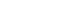bits on average, if we have a pre-shared mapping. As long as we use the mapping multiple times it amortizes the initial cost of sharing the mapping, so we're going to ignore it from here out. It's also worthwhile to keep this limit in mind as a max threshold for "reasonable;" we could easily create an encoding that is worse than this, which means that we've failed quite spectacularly at our job.

But, if there are additional constraints on which symbols appear, we should probably be able to do better. Consider the extreme situation where 95% of cars produced are red, 3% blue, and only 1% each for yellow and green. If I needed to transmit color descriptions for my factory's production of 10,000 vehicles, using the earlier scheme I'd need exactly 20,000 bits to do so by stringing together all of the colors in a single sequence. But, given that by the law of large numbers, I can expect roughly 9,500 cars to be red, so what if I use a different code, where red is assigned the bit string 0, blue is assigned 10, yellow is assigned 110, and green 111? Even though the representation for two of the colors is a bit longer in this scheme, the total average encoding length for a lot of 10,000 cars decreases to 10,700 bits (1*9500 + 2*300 + 3*100 + 3*100), almost an improvement of 50%! This suggests that the probabilities for each symbol should impact the compression mapping, because if some symbols are more common than others, we can make them shorter in exchange for making less common symbols longer and expect the average length of a message made from many symbols to decrease.

So, with that in mind, the next logical question is, how well can we do by adapting our compression scheme to the probability distribution for our set of symbols? And how do we find the mapping that achieves this best amount of compression? Consider a sequence of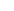independent, identically distributed symbols taken from some source with known probability mass function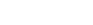, withtotal symbols for which the PMF is nonzero. If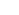is the number of times that theth symbol in the alphabet appears in the sequence, then by the law of large numbers we know that for largeit converges almost surely to a specific value: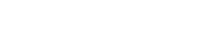.

In order to obtain an estimate of the best possible compression rate, we will use the threshold for reasonable compression identified earlier: it should, on average, take no more than approximatelybits to represent a value from a set, so by finding the number of possible sequences, we can bound how many bits it would take to describe them. A further consequence of the law of large numbers is that because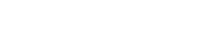we also have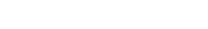. This means that we can expect the set of possible sequences to contain only the possible permutations of a sequence containingrealizations of each symbol. The probability of a specific sequence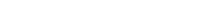can be expanded using the independence of each position and simplified by grouping like symbols in the resulting product:We still need to find the size of the setin order to find out how many bits we need. However, the probability we found above doesn't depend on the specific permutation, so it is the same for every element of the set and thus the distribution of sequences within the set is uniform. For a uniform distribution over a set of size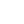, the probability of a specific element is, so we can substitute the above probability for any element and expand in order to find out how many bits we need for a string of length:Frequently, we're concerned with the number of bits required per symbol in the source sequence, so we dividebyto find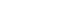, a quantity known as the entropy of the source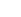, which has PMF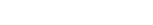:The entropy,, is important because it establishes the lower bound on the number of bits that is required, on average, to accurately represent a symbol taken from the corresponding sourcewhen encoding a large number of symbols.is non-negative, but it is not restricted to integers only; however, achieving less than one bit per symbol requires multiple neighboring symbols to be combined and encoded in groups, similarly to the method used above to obtain the expected bit rate. Unfortunately, that process cannot be used in practice for compression, because it requires enumerating an exponential number of strings (as a function of a variable tending towards infinity) in order to assign each sequence to a bit representation. Luckily, two very common, practical methods exist, Huffman Coding and Arithmetic Coding, that are guaranteed to achieve optimal performance.

For the car example mentioned earlier, the entropy works out to about 0.35 bits, which means there is significant room for improvement over the symbol-wise mapping I suggested, which only achieved a rate of 1.07 bits per symbol, but it would require grouping multiple car colors into a compound symbol, which quickly becomes tedious when working by hand. It is kind of amazing that using only ~3,500 bits, we could communicate the car colors that naively required 246,400 bits (=30,800 bytes) by encoding each letter of the English word with a single byte.also has other applications, including gambling, investing, lossy compression, communications systems, and signal processing, where it is generally used to establish the bounds for best- or worst-case performance. If you're interested in a more rigorous definition of entropy and a more formal derivation of the bounds on lossless compression, plus some applications, I'd recommend reading Claude Shannon's original paper on the subject, which effectively created the field of information theory.